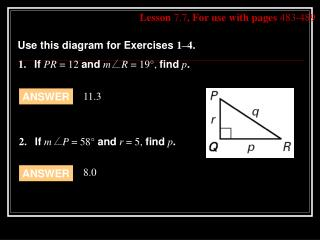DownloadDownload PresentationLesson 7.7 , For use with pages 483-489

# Lesson 7.7 , For use with pages 483-489

Télécharger la présentation## Lesson 7.7 , For use with pages 483-489

- - - - - - - - - - - - - - - - - - - - - - - - - - - E N D - - - - - - - - - - - - - - - - - - - - - - - - - - -
##### Presentation Transcript

1. 1.If PR = 12 andm R = 19°, findp. 11.3 ANSWER 2. Ifm P = 58° andr = 5, findp. 8.0 ANSWER Lesson 7.7, For use with pages 483-489 Use this diagram for Exercises 1–4.

2. 3.If mP = 60°, andp = 9 , findq. 10.4 ANSWER 14.4 ANSWER Lesson 7.7, For use with pages 483-489 Use this diagram for Exercises 1–4. 4. Ifr = 8 andp = 12, findq.

3. 7.7 Solve Right Triangles

4. To solve a right triangle: • Find the measures of all its sides • Find the measures of all its angles.

5. You will need to know one of the following: • Two side lengths • One side length and the measure of one acute angle

6. Inverse Trig Ratios – when you 2 side lengths

7. Example 1: • Use the calculator to approximate the measure of <Q to the nearest tenth of a degree.

8. Example 2: Let <C be an acute angle in a right triangle. Use the calculator to approximate the measure of <C to the nearest tenth of a degree. • sin C = 0.24 • cos C = 0.37 • sin C = 0.76 • cos C = 0.17

9. Example 3: • Solve the right triangle formed by the water slide shown in the figure. Round decimal answers to the nearest tenth.

10. Example 4: • Solve the right triangle. Round decimal answers to the nearest tenth.

11. Example 5: • A road rises 10 feet in a horizontal distance of 200 feet. What is the angle of inclination?

12.  Homework  • Pgs. 485 – 486 (3, 4, 6 – 8, 10 – 18 evens)